## Saturday, August 27, 2016

### Function to find all possible rotations of the nodes of a tree

The following is a function to find all possible rotated topologies for a bifurcating tree. Note that the number of such trees is very large! In particular, it should be 2m for m internal nodes. This is the same as N - 1 for N if the tree is perfectly bifurcating.

This quantity is thus:

``````2^(5-1) ## for five taxa
``````
``````##  16
``````
``````2^(8-1) ## for eight taxa
``````
``````##  128
``````
``````2^(10-1) ## for 10 taxa
``````
``````##  512
``````
``````2^(20-1) ## for twenty taxa
``````
``````##  524288
``````
``````2^(100-1) ## for 100 taxa
``````
``````##  6.338253e+29
``````

You get the idea.

Obviously, we should only do this for relatively small trees!

Here is the function:

``````library(phytools)
## function to compute all rotations
allRotations<-function(tree){
if(!is.binary.tree(tree)){
was.binary<-FALSE
if(is.null(tree\$edge.length)){
tree<-compute.brlen(tree)
tree<-multi2di(tree)
} else was.binary<-TRUE
nodes<-1:tree\$Nnode+Ntip(tree)
trees<-vector(mode="list",length=2^length(nodes))
ii<-2
trees[]<-tree
for(i in 1:length(nodes)){
N<-ii-1
for(j in 1:N){
trees[[ii]]<-rotate(trees[[j]],nodes[i])
ii<-ii+1
}
}
if(!was.binary){
trees<-lapply(trees,di2multi)
function(x){
x\$edge.length<-NULL
x
})
}
class(trees)<-"multiPhylo"
trees
}
``````

Let's try it:

``````## five taxa
tree<-rtree(n=5,br=NULL)
trees<-allRotations(tree)
trees
``````
``````## 16 phylogenetic trees
``````
``````par(mfrow=c(4,4))
plotTree(trees,mar=rep(1.1,4))
``````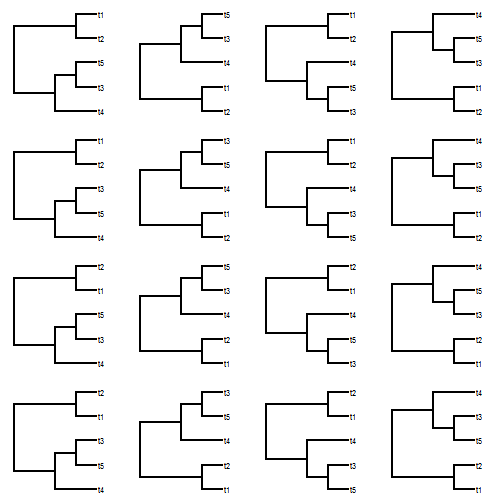``````## six taxa
trees<-allRotations(rtree(n=6,br=NULL))
trees
``````
``````## 32 phylogenetic trees
``````
``````par(mfrow=c(8,4))
plotTree(trees,mar=rep(1.1,4),ylim=c(0.8,6.2))
``````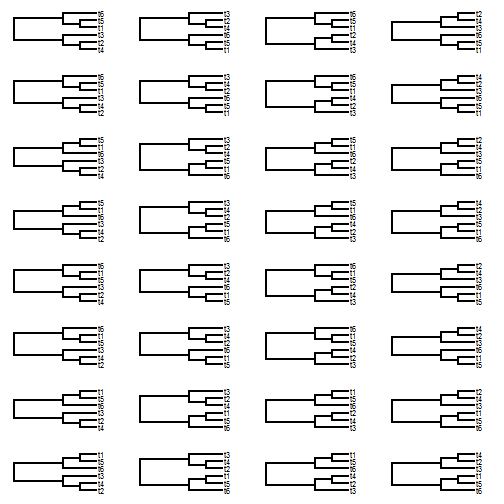``````## ten taxa
trees<-allRotations(rtree(n=10,br=NULL))
par(mfrow=c(32,16))
plotTree(trees,lwd=1,fsize=0.1)
``````### Finding the association between two trees when some taxa are missing or when each host lineage matches with a clade of parasites

I've been playing testing the optimization method for tip matching in the `cophylo` method for co-phylogenetic plotting. Although a formal proof is lacking (& not forthcoming!), I have shown via simulation that when trees match precisely in their topology and labels, `cophylo` can successfully find this matching - even for large trees that are completely scrambled a priori.

This also seems to be true when some lineages are missing from one or the other tree - for instance when cospeciation is perfect, but some taxa have been lost due to extinction or missing taxa; and when cospeciation is perfect, but host lineages might possess multiple, divergent parasite races, themselves forming a monophyletic group - such as when 'speciation' for the parasite lineages occurs more rapidly than in the hosts.

Below, I demonstrate both of these cases.

First, with some missing lineages. Let's start by simulating:

``````library(phytools)
## simulate matching trees
t1<-t2<-rtree(n=100)
## rotate nodes randomly in one of the trees
for(i in 1:100) t2<-rotateNodes(t2,sample(1:t2\$Nnode+Ntip(t2),1))
## drop lineages from each tree at random
t1<-drop.tip(t1,sample(t1\$tip.label,40))
t2<-drop.tip(t2,sample(t2\$tip.label,30))
## these trees are fully scrambled!
plot(cophylo(t1,t2,rotate=FALSE),fsize=0.7)
``````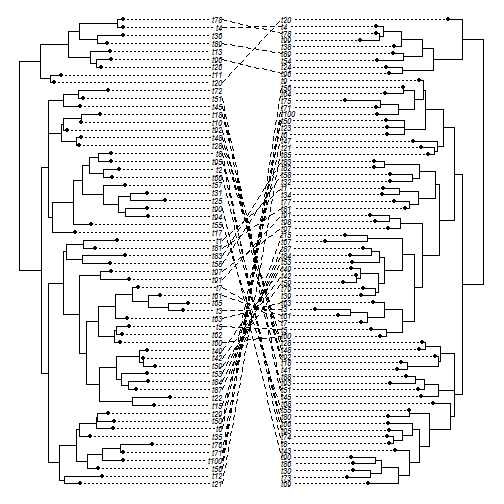``````## match them by rotating nodes
obj<-cophylo(t1,t2)
``````
``````## Rotating nodes to optimize matching...
## Done.
``````
``````obj
``````
``````## Object of class "cophylo" containing:
##
## (1) 2 (possibly rotated) phylogenetic trees in an object of class "multiPhylo".
##
## (2) A table of associations between the tips of both trees.
``````
``````plot(obj,fsize=0.7)
``````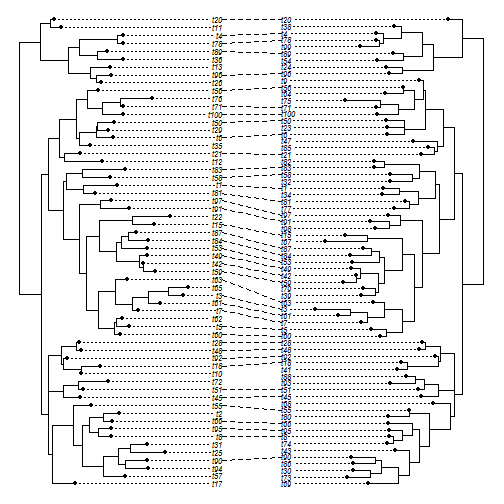Next, let's imagine a case in which cospeciation is perfect, but in which each host species matches with a clade of parasites (for instance). We can simulate this by first generating host & parasite trees that match, then slicing the host tree at a time point (say, when 26 lineages are extant). Next we compute all the descendants from that point in each subtree. These are our parasite clades.

``````## simulate trees
host<-parasite<-pbtree(n=100,scale=10)
## slice to get all subtrees
LTT<-ltt(host,plot=FALSE)
t<-LTT\$times[which(LTT\$ltt==26)]
subs<-treeSlice(parasite,t,trivial=TRUE)
hosts<-sapply(subs,function(x) x\$tip.label)
host<-drop.tip(host,setdiff(host\$tip.label,hosts))
host\$tip.label<-LETTERS
for(i in 1:Ntip(host)){
if(i==1) assoc<-cbind(rep(host\$tip.label[i],Ntip(subs[[i]])),
subs[[i]]\$tip.label)
else {
assoc<-rbind(assoc,
cbind(rep(host\$tip.label[i],Ntip(subs[[i]])),
subs[[i]]\$tip.label))
}
}
host
``````
``````##
## Phylogenetic tree with 26 tips and 25 internal nodes.
##
## Tip labels:
##  A, B, C, D, E, F, ...
##
## Rooted; includes branch lengths.
``````
``````parasite
``````
``````##
## Phylogenetic tree with 100 tips and 99 internal nodes.
##
## Tip labels:
##  t1, t4, t50, t51, t23, t91, ...
##
## Rooted; includes branch lengths.
``````
``````assoc
``````
``````##        [,1] [,2]
##   [1,] "A"  "t1"
##   [2,] "B"  "t4"
##   [3,] "C"  "t50"
##   [4,] "C"  "t51"
##   [5,] "D"  "t23"
##   [6,] "D"  "t91"
##   [7,] "D"  "t92"
##   [8,] "E"  "t20"
##   [9,] "E"  "t21"
##  [10,] "F"  "t61"
##  [11,] "F"  "t62"
##  [12,] "F"  "t15"
##  [13,] "G"  "t14"
##  [14,] "G"  "t16"
##  [15,] "G"  "t38"
##  [16,] "G"  "t39"
##  [17,] "G"  "t30"
##  [18,] "H"  "t2"
##  [19,] "I"  "t36"
##  [20,] "I"  "t37"
##  [21,] "I"  "t33"
##  [22,] "I"  "t34"
##  [23,] "J"  "t8"
##  [24,] "J"  "t22"
##  [25,] "J"  "t73"
##  [26,] "J"  "t74"
##  [27,] "J"  "t48"
##  [28,] "K"  "t5"
##  [29,] "L"  "t6"
##  [30,] "M"  "t55"
##  [31,] "M"  "t56"
##  [32,] "N"  "t99"
##  [33,] "N"  "t100"
##  [34,] "N"  "t64"
##  [35,] "N"  "t52"
##  [36,] "N"  "t17"
##  [37,] "N"  "t40"
##  [38,] "N"  "t41"
##  [39,] "O"  "t19"
##  [40,] "O"  "t68"
##  [41,] "O"  "t69"
##  [42,] "O"  "t18"
##  [43,] "P"  "t25"
##  [44,] "P"  "t43"
##  [45,] "P"  "t44"
##  [46,] "P"  "t70"
##  [47,] "P"  "t71"
##  [48,] "P"  "t63"
##  [49,] "P"  "t11"
##  [50,] "P"  "t10"
##  [51,] "P"  "t9"
##  [52,] "Q"  "t13"
##  [53,] "Q"  "t46"
##  [54,] "Q"  "t47"
##  [55,] "Q"  "t42"
##  [56,] "R"  "t28"
##  [57,] "R"  "t29"
##  [58,] "S"  "t49"
##  [59,] "S"  "t89"
##  [60,] "S"  "t90"
##  [61,] "T"  "t26"
##  [62,] "T"  "t27"
##  [63,] "U"  "t45"
##  [64,] "U"  "t79"
##  [65,] "U"  "t80"
##  [66,] "U"  "t97"
##  [67,] "U"  "t98"
##  [68,] "U"  "t12"
##  [69,] "V"  "t7"
##  [70,] "W"  "t57"
##  [71,] "W"  "t58"
##  [72,] "W"  "t59"
##  [73,] "W"  "t81"
##  [74,] "W"  "t82"
##  [75,] "W"  "t60"
##  [76,] "W"  "t32"
##  [77,] "X"  "t24"
##  [78,] "X"  "t75"
##  [79,] "X"  "t76"
##  [80,] "X"  "t77"
##  [81,] "X"  "t78"
##  [82,] "X"  "t65"
##  [83,] "X"  "t66"
##  [84,] "X"  "t67"
##  [85,] "X"  "t95"
##  [86,] "X"  "t96"
##  [87,] "Y"  "t53"
##  [88,] "Y"  "t54"
##  [89,] "Y"  "t35"
##  [90,] "Y"  "t93"
##  [91,] "Y"  "t94"
##  [92,] "Y"  "t85"
##  [93,] "Y"  "t86"
##  [94,] "Y"  "t72"
##  [95,] "Y"  "t83"
##  [96,] "Y"  "t84"
##  [97,] "Y"  "t87"
##  [98,] "Y"  "t88"
##  [99,] "Y"  "t31"
## [100,] "Z"  "t3"
``````
``````## scramble order of parasites
for(i in 1:100) parasite<-rotateNodes(parasite,sample(1:parasite\$Nnode+
Ntip(parasite),1))
## they are fully scrambled
plot(cophylo(host,parasite,assoc=assoc,rotate=FALSE),
fsize=c(1,0.5))
``````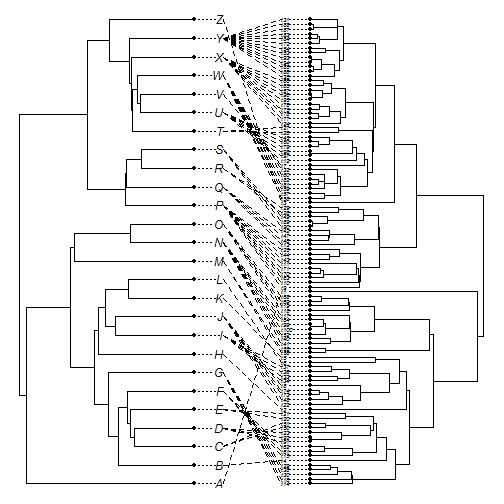``````## rotate to optimize matching
obj<-cophylo(host,parasite,assoc=assoc)
``````
``````## Rotating nodes to optimize matching...
## Done.
``````
``````plot(obj,fsize=c(1,0.5),link.type="curved")
``````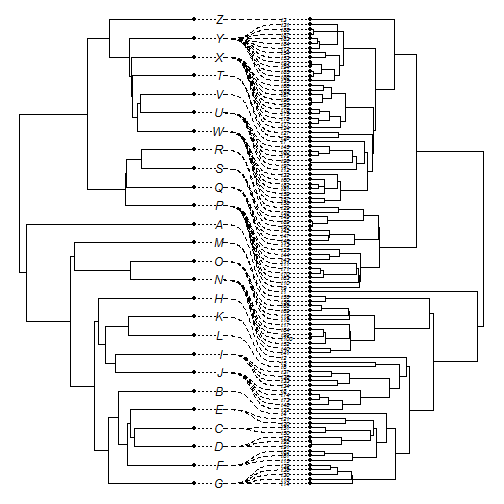Pretty neat, right?